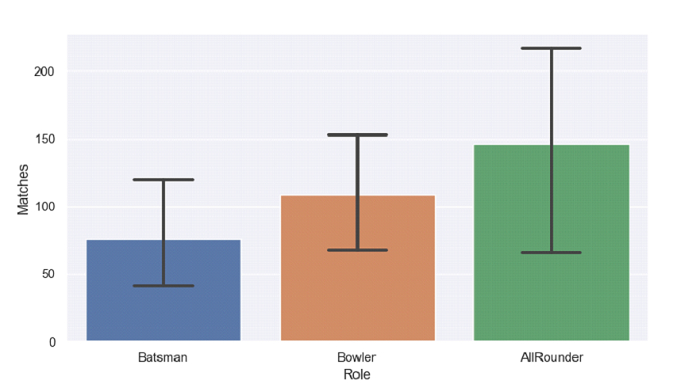# Python Pandas - Draw a bar plot and set a cap to the error bars with Seaborn

Bar Plot in Seaborn is used to show point estimates and confidence intervals as rectangular bars. The seaborn.barplot() is used. Set caps to the error bars using the capsize parameter.

Let’s say the following is our dataset in the form of a CSV file − Cricketers2.csv

At first, import the required libraries −

import seaborn as sb
import pandas as pd
import matplotlib.pyplot as plt

Load data from a CSV file into a Pandas DataFrame −

dataFrame = pd.read_csv("C:\Users\amit_\Desktop\Cricketers2.csv")


Set caps to the error bars using the capsize parameter −

sb.barplot(x=dataFrame["Role"], y=dataFrame["Matches"], capsize=.3)

## Example

Following is the code −

import seaborn as sb
import pandas as pd
import matplotlib.pyplot as plt

# Load data from a CSV file into a Pandas DataFrame

sb.set_theme(style="darkgrid")

# bar plot
# setting caps to the error bars using the capsize parameter
sb.barplot(x=dataFrame["Role"], y=dataFrame["Matches"], capsize=.3)

# display
plt.show()

## Output

This will produce the following output −